## How to Calculate and Solve for Viscosity | Non Polar Gases | Transport Phenomena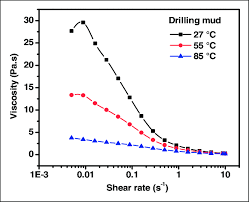The image above represents viscosity.

To compute for viscosity, four essential parameters are needed and these parameters are Gram Molecular Weight (M), Temperature (Kelvin) (T), Characteristic Diameter (σ) and Collision Integral of Chapman Enskog Theory ( ηn).

The formula for calculating viscosity:

η = 2.67E-5√(MT) / σ².ηn

Where:

η = Viscosity | Non Polar Gases
M = Gram Molecular Weight
T = Temperature (Kelvin)
λ = Characteristic Diameter
ηn = Collision Integral of Chapman Enskog Theory

Let’s solve an example;
Find the viscosity when the gram molecular weight is 10, the temperature is 12, the characteristic diameter is 14 and the collision integral of chapman enskog theory is 16.

This implies that;

M = Gram Molecular Weight = 10
T = Temperature (Kelvin) = 12
λ = Characteristic Diameter = 14
ηn = Collision Integral of Chapman Enskog Theory = 16

η = 2.67E-5√(MT) / σ².ηn
η = 2.67E-5√((10)(12)) / (14)².(16)
η = 2.67E-5√(120) / (196).(16)
η = 2.67E-5(10.954) / 3136
η = 0.000292 / 3136
η = 9.326e-8

Therefore, the viscosity is 9.326e-8 m²/s.

## How to Calculate and Solve for Newton’s Laws of Viscosity of Gases | Transport Phenomena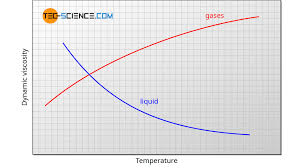The image above represents newton’s laws of viscosity of gases.

To compute for newton’s laws of viscosity of gases, four essential parameters are needed and these parameters are Number of Molecules (m), Boltzmann’s Constant (KB), Temperature (T) and Pipe Diameter (d).

The formula for calculating newton’s laws of viscosity of gases:

η = 2(mKBT)0.5 / 1.5.d²

Where:

η = Newton’s Law of Viscosity of Gases
m = Number of Molecules
KB = Boltzmann’s Constant
T = Temperature
d = Pipe Diameter

Let’s solve an example;
Find the newton’s law of viscosity of gases when the number of molecules is 14, the boltzmann’s constant is 1.380E-23, the temperature is 10 and the pipe diameter is 21.

This implies that;

m = Number of Molecules = 14
KB = Boltzmann’s Constant = 1.380E-23
T = Temperature = 10
d = Pipe Diameter = 21

η = 2(mKBT)0.5 / 1.5.d²
η = 2((14)(1.380e-23)(10))0.5 / 1.5.(21)²
η = 2(1.93e-21)0.5 / (16.70).(441)
η = 2(4.396e-11) / 7366.89
η = 8.79e-11 / 7366.89
η = 1.19e-14

Therefore, the newton’s law of viscosity of gases is 1.19e-14 m²/s.

## How to Calculate and Solve for Mean Free Path | Transport Phenomena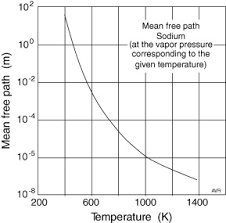The image above represents mean free path.

To compute for mean free path, two essential parameters are needed and these parameters are Pipe Diameter (D) and Number of Molecules (m).

The formula for calculating mean free path:

λ = 1 / √(2)π.D²m

Where:

λ = Mean Free Path
D = Pipe Diameter
m = Number of Molecules

Let’s solve an example;
Find the mean free path when the pipe diameter is 35 and the number of molecules is 7.

This implies that;

D = Pipe Diameter = 35
m = Number of Molecules = 7

λ = 1 / √(2)π.D²m
λ = 1 / √(2)π..(35)²(7)
λ = 1 / (4.442).(1225)(7)
λ = 1 / (4.442).(8575)
λ = 1 / 38097.721
λ = 0.0000262

Therefore, the mean free path is 0.0000262.

## How to Calculate and Solve for Average Speed of Gases | Transport Phenomena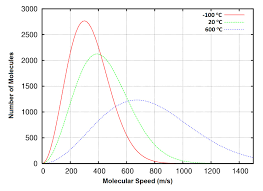The image above represents average speed of gases.

To compute for average speed of gases, three essential parameters are needed and these parameters are Boltzmann’s Constant (KB), Absolute Temperature (T) and Number of Molecules (m).

The formula for calculating average speed of gases:

v’ = (8KBT / πm)0.5

Where:

v’ = Average Speed of Gases
KB = Boltzmann’s Constant
T = Absolute Temperature
m = Number of Molecules

Let’s solve an example;
Find the average speed of gases when the boltzmann’s constant is 1.380E-23, the absolute temperature is 12 and the number of molecules is 2.

This implies that;

KB = Boltzmann’s Constant = 1.380E-23
T = Absolute Temperature = 12
m = Number of Molecules = 2

v’ = (8KBT / πm)0.5
v’ = (8(1.3806e-23)(12) / 3.142(2))0.5
v’ = (1.325e-21 / 6.2831)0.5
v’ = (2.109e-22)0.5
v’ = 1.45e-11

Therefore, the average speed of gases is 1.45e-11 m/s.

## How to Calculate and Solve for Kinematic Viscosity | Transport Phenomena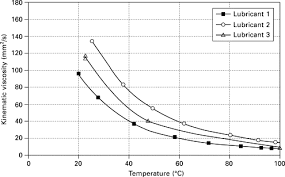The image above represents kinematic viscosity.

To compute for kinematic viscosity, two essential parameters are needed and these parameters are Viscosity (η) and Density (ρ).

The formula for calculating kinematic viscosity:

V = η / ρ

Where:

V = Kinematic Viscosity
η = Viscosity
ρ = Density

Let’s solve an example;
Find the kinematic viscosity when the viscosity is 44 and the density is 11.

This implies that;

η = Viscosity = 44
ρ = Density = 11

V = η / ρ
V = 44 / 11
V = 4

Therefore, the kinematic viscosity is 4 m²/s.

Calculating the Viscosity when the Kinematic Viscosity and the Density is Given.

η = V x ρ

Where:

η = Viscosity
V = Kinematic Viscosity
ρ = Density

Let’s solve an example;
Find the viscosity when the kinematic viscosity is 10 and the density is 5.

This implies that;

V = Kinematic Viscosity = 10
ρ = Density = 5

η = V x ρ
η = 10 x 5
η = 50

Therefore, the viscosity is 50.

## How to Calculate and Solve for Average Fluid Velocity | Transport Phenomena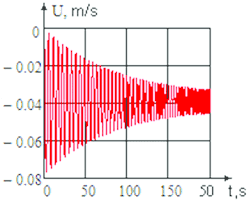The image above represents average fluid velocity.

To compute for average fluid velocity, two essential parameters are needed and these parameters are Volume Flow Rate, Q and Pipe Diameter (D).

The formula for calculating average fluid velocity:

v’ = 4Q / πD²

Where:

v’ = Average Fluid Velocity
Q = Volume Flow Rate
D = Pipe Diameter

Let’s solve an example;
With a volume flow rate of 42 and a pipe diameter of 8. Find the average fluid velocity?

This implies that;

Q = Volume Flow Rate = 42
D = Pipe Diameter = 8

v’ = 4Q / πD²
v’ = 4(42) / π(8)²
v’ = 168 / π(64)
v’ = 168 / 201.06
v’ = 0.835

Therefore, the average fluid velocity is 0.835 m/s.

Calculating the Volume Flow Rate when the Average Fluid Velocity and the Pipe Diameter is Given.

Q = v’ x πD² / 4

Where:

Q = Volume Flow Rate
v’ = Average Fluid Velocity
D = Pipe Diameter

Let’s solve an example;
Find the volume flow rate when the average fluid velocity is 20 and the pipe diameter is 8.

This implies that;

v’ = Average Fluid Velocity = 20
D = Pipe Diameter = 8

Q = v’ x πD² / 4
Q = 20 x π(8)² / 4
Q = 20 x 201.06 / 4
Q = 4021.2 / 4
Q = 1005.3

Therefore, the volume flow rate is 1005.3.

## How to Calculate and Solve for Reynold’s Number | Transport Phenomena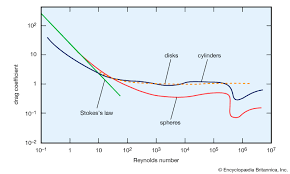The image above represents reynold’s number.

To compute for reynold’s number, three essential parameters are needed and these parameters are Average Fluid Velocity (v’), Pipe Diameter (D) and Kinematic Viscosity (V).

The formula for calculating the reynold’s number:

Re = V’.D / V

Where:

Re = Reynold’s Number
v’ = Average Fluid Velocity
D = Pipe Diameter
V = Kinematic Viscosity

Let’s solve an example;
Find the reynold’s number when the average fluid velocity is 21, the pipe diameter is 12 and the kinematic viscosity is 7.

This implies that;

v’ = Average Fluid Velocity = 21
D = Pipe Diameter = 12
V = Kinematic Viscosity = 7

Re = V’.D / V
Re = (21).(12) / 7
Re = 252 / 7
Re = 36

Therefore, the reynold’s number is 36.

Calculating the Average Fluid Velocity when the Reynold’s Number, the Pipe Diameter and the Kinematic Viscosity is Given.

v’ = Re x V / D

Where:

v’ = Average Fluid Velocity
Re = Reynold’s Number
D = Pipe Diameter
V = Kinematic Viscosity

Let’s solve an example;
Find the average fluid velocity when the reynold’s number is 12, the pipe diameter is 4 and the kinematic viscosity is 2.

This implies that;

Re = Reynold’s Number = 12
D = Pipe Diameter = 4
V = Kinematic Viscosity = 2

v’ = Re x V / D
v’ = 12 x 2 / 4
v’ = 24 / 4
v’ = 6

Therefore, the average fluid velocity is 6.

## How to Calculate and Solve for Breakaway Pressure | Energy Transport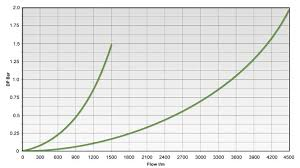The image above represents breakaway pressure.

To compute for breakaway pressure, three essential parameters are needed and these parameters are Temperature (T), Average Particle Diameter (Dp) and Mean Diameter of Gas Particle (d).

The formula for calculating breakaway pressure:

Pb = 4.32 x 10-21(T / Dpd²)

Where:

Pb = Breakaway Pressure
T = Temperature
Dp = Average Particle Diameter
d = Mean Diameter of Gas Particle

Let’s solve an example;
Find the breakaway pressure when the temperature is 7, the average particle diameter is 14 and the mean diameter of gas particle is 3.

This implies that;

T = Temperature = 7
Dp = Average Particle Diameter = 14
d = Mean Diameter of Gas Particle = 3

Pb = 4.32 x 10-21(T / Dpd²)
Pb = 4.32 x 10-21(7 / (14)(3)²)
Pb = 4.32 x 10-21(7 / (14)(9))
Pb = 4.32 x 10-21(7 / 126)
Pb = 4.32 x 10-21(0.055)
Pb = 2.4e-22

Therefore, the breakaway pressure is 2.4e-22 atm.

## How to Calculate and Solve for Speed of Sound in Molten Metal | Energy Transport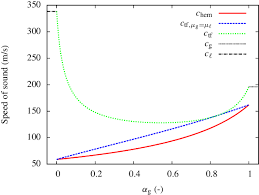The image above represents speed of sound in molten metal.

To compute for speed of sound in molten metal, two essential parameters are needed and these parameters are Melting Temperature (Tm) and Molecular Weight (M).

The formula for calculating speed of sound in molten metal:

us = 16(Tm / M)0.5

Where:

us = Speed of Sound in Molten Metal
Tm = Melting Temperature
M = Molecular Weight

Let’s solve an example;
Find the speed of sound in molten metal when the melting temperature is 24 and the molecular weight is 12.

This implies that;

Tm = Melting Temperature = 24
M = Molecular Weight = 12

us = 16(Tm / M)0.5
us = 16(24 / 12)0.5
us = 16(2)0.5
us = 16(1.414)
us = 22.627

Therefore, the speed of sound in molten metal is 22.627 m/s.

## How to Calculate and Solve for Speed of Sound throughout Liquid | Energy Transport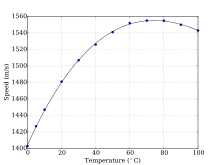The image above represents speed of sound throughout liquid.

To compute for speed of sound throughout liquid, four essential parameters are needed and these parameters are Heat Capacity at Constant Pressure (cp), Heat Capacity at Constant Volume (cv), Density (ρ) and Compressibility (β).

The formula for calculating the speed of sound throughout liquid:

vs = (cp / cvρβ)0.5

Where:

vs = Speed of Sound throughout Liquid
cp = Heat Capacity at Constant Pressure
cv = Heat Capacity at Constant Volume
ρ = Density
β = Compressibility

Let’s solve an example;
Find the speed of sound throughout liquid when the heat capacity at constant pressure is 12, the heat capacity at constant volume is 10, the density is 20 and the compressibility is 14.

This implies that;

cp = Heat Capacity at Constant Pressure = 12
cv = Heat Capacity at Constant Volume = 10
ρ = Density = 20
β = Compressibility = 14

vs = (cp / cvρβ)0.5
vs = (10 / (10)(20)(14))0.5
vs = (10 / 2800)0.5
vs = (0.00428)0.5
vs = 0.065

Therefore, the speed of sound throughout liquid is 0.065 m/s.